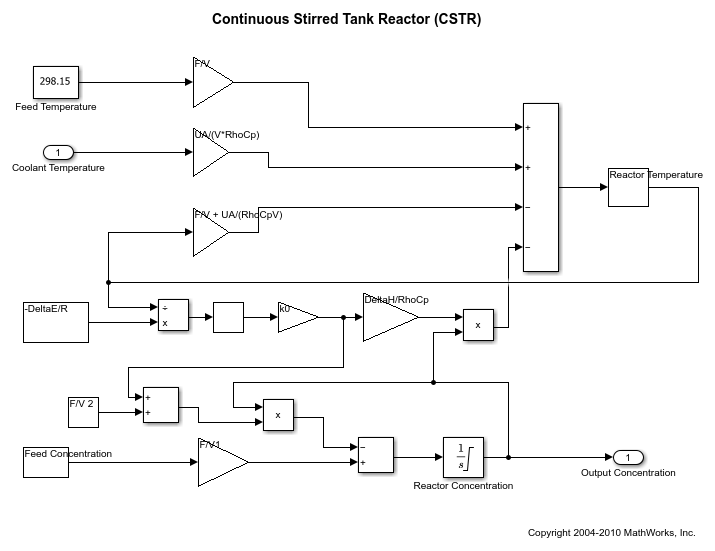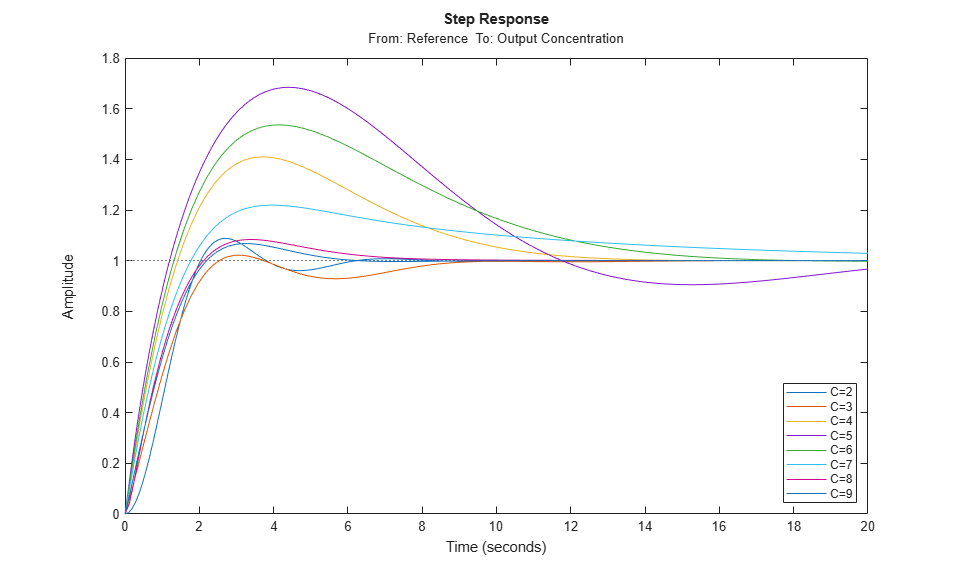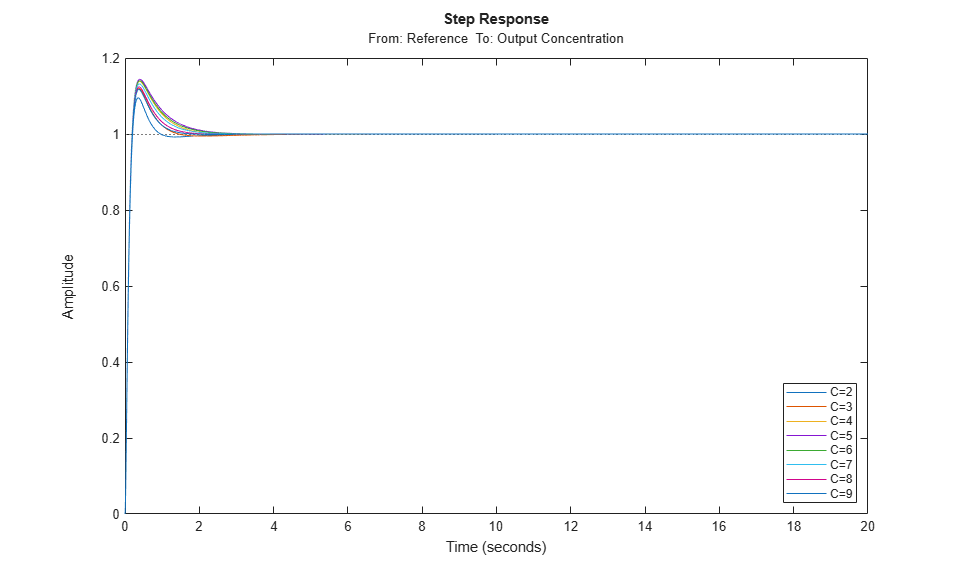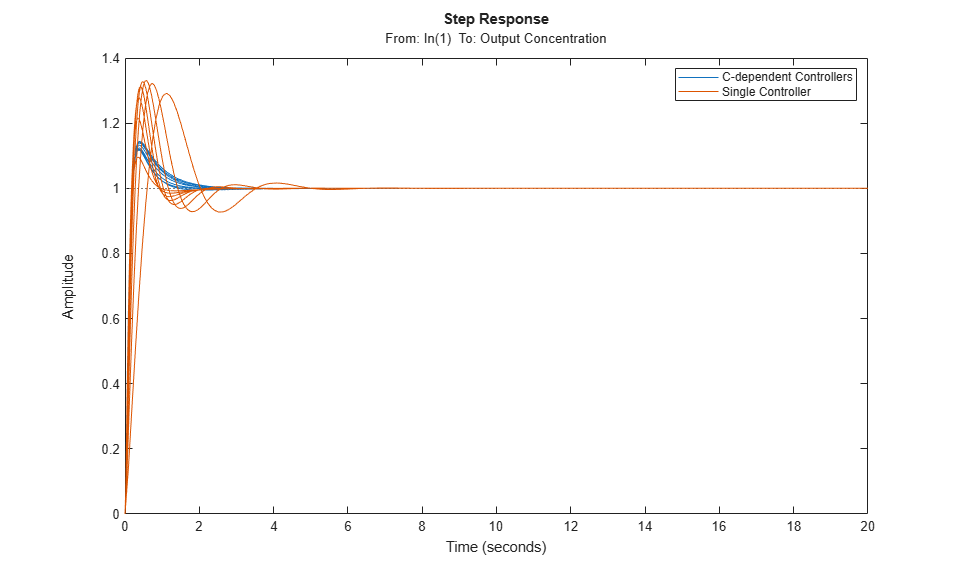Documentation

### This is machine translation

Mouseover text to see original. Click the button below to return to the English version of the page.

Note: This page has been translated by MathWorks. Click here to see
To view all translated materials including this page, select Country from the country navigator on the bottom of this page.

## Design Family of PID Controllers for Multiple Operating Points

This example shows how to design an array of PID controllers for a nonlinear plant in Simulink that operates over a wide range of operating points.

### Open Plant Model

The plant is a continuous stirred tank reactor (CSTR) that operates over a wide range of operating points. A single PID controller can effectively use the coolant temperature to regulate the output concentration around a small operating range that the PID controller is designed for. However, since the plant is a strongly nonlinear system, control performance degrades if operating point changes significantly. The closed-loop system can even become unstable.

Open the CSTR plant model.

```mdl = 'scdcstrctrlplant'; open_system(mdl) ```For more information on this system, see .

### Introduction to Gain Scheduling

A common approach to solve the nonlinear control problem is using gain scheduling with linear controllers. Generally speaking, designing a gain scheduling control system takes four steps:

1. Obtain a plant model for each operating region. The usual practice is to linearize the plant at several equilibrium operating points.

2. Design a family of linear controllers, such as PID controllers, for the plant models obtained in the previous step.

3. Implement a scheduling mechanism such that the controller coefficients, such as PID gains, are changed based on the values of the scheduling variables. Smooth (bumpless) transfer between controllers is required to minimize disturbance to plant operation.

4. Assess control performance with simulation.

For more information on gain scheduling, see .

This example focuses on designing a family of PID controllers for the nonlinear CSTR plant.

### Obtain Linear Plant Models for Multiple Operating Points

The output concentration `C` is used to identify different operating regions. The CSTR plant can operate at any conversion rate between a low conversion rate (`C` = `9`) and a high conversion rate (`C` = `2`). In this example, divide the operating range into eight regions represented by `C` = `2` through `9`.

Specify the operating regions.

```C = [2 3 4 5 6 7 8 9]; ```

Create an array of default operating point specifications.

```op = operspec(mdl,numel(C)); ```

Initialize the operating point specifications by specifying that the output concentration is a known value, and specifying the output concentration value.

```for ct = 1:numel(C) op(ct).Outputs.Known = true; op(ct).Outputs.y = C(ct); end ```

Compute the equilibrium operating points corresponding to the values of `C`.

```opoint = findop(mdl,op,findopOptions('DisplayReport','off')); ```

Linearize the plant at these operating points.

```Plants = linearize(mdl,opoint); ```

Since the CSTR plant is nonlinear, the linear models display different characteristics. For example, plant models with high and low conversion rates are stable, while the others are not.

```isstable(Plants,'elem')' ```
```ans = 1x8 logical array 1 1 0 0 0 0 1 1 ```

### Design PID Controllers for the Plant Models

To design multiple PID controllers in batch, use the `pidtune` function. The following command generates an array of PID controllers in parallel form. The desired open-loop crossover frequency is at `1` rad/sec and the phase margin is the default value of `60` degrees.

```Controllers = pidtune(Plants,'pidf',pidtuneOptions('Crossover',1)); ```

Display the controller for `C` = `4`.

```Controllers(:,:,4) ```
```ans = 1 s Kp + Ki * --- + Kd * -------- s Tf*s+1 with Kp = -12.4, Ki = -1.74, Kd = -16, Tf = 0.00875 Continuous-time PIDF controller in parallel form. ```

To analyze the closed-loop responses for step setpoint tracking, first construct the closed-loop systems.

```clsys = feedback(Plants*Controllers,1); ```

Plot closed-loop responses

```figure hold on for ct = 1:length(C) % Select a system from the LTI array sys = clsys(:,:,ct); sys.Name = ['C=',num2str(C(ct))]; sys.InputName = 'Reference'; % Plot step response stepplot(sys,20); end legend('show','location','southeast') ```All the closed loops are stable, but the overshoots of the loops with unstable plants (`C` = `4`, through `7`) are too large. To improve the results, increase the target open-loop bandwidth to `10` rad/sec.

Design updated controllers for the unstable plant models.

```Controllers = pidtune(Plants,'pidf',10); ```

Display the controller for `C` = `4`.

```Controllers(:,:,4) ```
```ans = 1 s Kp + Ki * --- + Kd * -------- s Tf*s+1 with Kp = -283, Ki = -151, Kd = -128, Tf = 0.0183 Continuous-time PIDF controller in parallel form. ```

Construct the closed-loop systems, and plot the closed-loop step responses for the new controllers.

```clsys = feedback(Plants*Controllers,1); figure hold on for ct = 1:length(C) % Select a system from the LTI array sys = clsys(:,:,ct); set(sys,'Name',['C=',num2str(C(ct))],'InputName','Reference'); % Plot step response stepplot(sys,20); end legend('show','location','southeast') ```All the closed-loop responses are now satisfactory. For comparison, examine the response when you use the same controller at all operating points. Create another set of closed-loop systems, where each one uses the `C` = `2` controller, and plot their responses.

```clsys_flat = feedback(Plants*Controllers(:,:,1),1); figure stepplot(clsys,clsys_flat,20) legend('C-dependent Controllers','Single Controller') ```The array of PID controllers designed separately for each concentration gives considerably better performance than a single controller.

However, the closed-loop responses shown above are computed based on linear approximations of the full nonlinear system. To validate the design, implement the scheduling mechanism in your model using the PID Controller block.

Close the model.

```bdclose(mdl) ```

### References

 Seborg, D. E., T. F. Edgar, and D. A. Mellichamp. Process Dynamics and Control, 2nd Ed., Wiley, 2004, pp. 34-36.

 Rugh, W. J., and J. S. Shamma. "Research on gain scheduling." Automatica, Issue 36, 2000, pp. 1401-1425.

##### SupportGet trial now